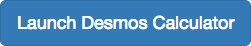## Operations on Square Roots

### Learning Outcomes

• Add and subtract square roots.
• Rationalize denominators.We can add or subtract radical expressions only when they have the same radicand and when they have the same radical type such as square roots. For example, the sum of $\sqrt{2}$ and $3\sqrt{2}$ is $4\sqrt{2}$. However, it is often possible to simplify radical expressions, and that may change the radicand. The radical expression $\sqrt{18}$ can be written with a $2$ in the radicand, as $3\sqrt{2}$, so $\sqrt{2}+\sqrt{18}=\sqrt{2}+3\sqrt{2}=4\sqrt{2}$.

### How To: Given a radical expression requiring addition or subtraction of square roots, solve.

Add $5\sqrt{12}+2\sqrt{3}$.

### Try It

Add $\sqrt{5}+6\sqrt{20}$.

Watch this video to see more examples of adding roots.

### Example: Subtracting Square Roots

Subtract $20\sqrt{72{a}^{3}{b}^{4}c}-14\sqrt{8{a}^{3}{b}^{4}c}$.

### Try It

Subtract $3\sqrt{80x}-4\sqrt{45x}$.

## Rationalize Denominators

When an expression involving square root radicals is written in simplest form, it will not contain a radical in the denominator. We can remove radicals from the denominators of fractions using a process called rationalizing the denominator.

We know that multiplying by 1 does not change the value of an expression. We use this property of multiplication to change expressions that contain radicals in the denominator. To remove radicals from the denominators of fractions, multiply by the form of 1 that will eliminate the radical.

For a denominator containing a single term, multiply by the radical in the denominator over itself. In other words, if the denominator is $b\sqrt{c}$, multiply by $\dfrac{\sqrt{c}}{\sqrt{c}}$.

For a denominator containing the sum or difference of a rational and an irrational term, multiply the numerator and denominator by the conjugate of the denominator, which is found by changing the sign of the radical portion of the denominator. If the denominator is $a+b\sqrt{c}$, then the conjugate is $a-b\sqrt{c}$.

### How To: Given an expression with a single square root radical term in the denominator, rationalize the denominator.

1. Multiply the numerator and denominator by the radical in the denominator.
2. Simplify.

### Example: Rationalizing a Denominator Containing a Single Term

Write $\dfrac{2\sqrt{3}}{3\sqrt{10}}$ in simplest form.

### Try It

Write $\dfrac{12\sqrt{3}}{\sqrt{2}}$ in simplest form.

### How To: Given an expression with a radical term and a constant in the denominator, rationalize the denominator.

1. Find the conjugate of the denominator.
2. Multiply the numerator and denominator by the conjugate.
3. Use the distributive property.
4. Simplify.

### Example: Rationalizing a Denominator Containing Two Terms

Write $\dfrac{4}{1+\sqrt{5}}$ in simplest form.

### Try It

Write $\dfrac{7}{2+\sqrt{3}}$ in simplest form.

## Contribute!

Did you have an idea for improving this content? We’d love your input.